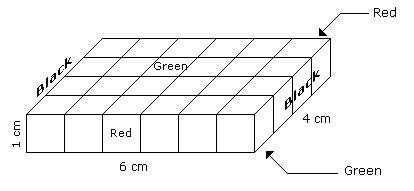# Verbal Reasoning - Cube and Cuboid

## Why Verbal Reasoning Cube and Cuboid?

In this section you can learn and practice Verbal Reasoning Questions based on "Cube and Cuboid" and improve your skills in order to face the interview, competitive examination and various entrance test (CAT, GATE, GRE, MAT, Bank Exam, Railway Exam etc.) with full confidence.

## Where can I get Verbal Reasoning Cube and Cuboid questions and answers with explanation?

IndiaBIX provides you lots of fully solved Verbal Reasoning (Cube and Cuboid) questions and answers with Explanation. Solved examples with detailed answer description, explanation are given and it would be easy to understand. All students, freshers can download Verbal Reasoning Cube and Cuboid quiz questions with answers as PDF files and eBooks.

## Where can I get Verbal Reasoning Cube and Cuboid Interview Questions and Answers (objective type, multiple choice)?

Here you can find objective type Verbal Reasoning Cube and Cuboid questions and answers for interview and entrance examination. Multiple choice and true or false type questions are also provided.

## How to solve Verbal Reasoning Cube and Cuboid problems?

You can easily solve all kind of Verbal Reasoning questions based on Cube and Cuboid by practicing the objective type exercises given below, also get shortcut methods to solve Verbal Reasoning Cube and Cuboid problems.

### Exercise :: Cube and Cuboid - Cube and Cuboid 1

The following questions are based on the information given below:

1. A cuboid shaped wooden block has 6 cm length, 4 cm breadth and 1 cm height.
2. Two faces measuring 4 cm x 1 cm are coloured in black.
3. Two faces measuring 6 cm x 1 cm are coloured in red.
4. Two faces measuring 6 cm x 4 cm are coloured in green.
5. The block is divided into 6 equal cubes of side 1 cm (from 6 cm side), 4 equal cubes of side 1 cm(from 4 cm side).
1.

How many cubes having red, green and black colours on at least one side of the cube will be formed ?

 A. 16 B. 12 C. 10 D. 4

Explanation:Such cubes are related to the corners of the cuboid. Since the number of corners of the cuboid is 4.

Hence, the number of such small cubes is 4.

2.

How many small cubes will be formed ?

 A. 6 B. 12 C. 16 D. 24

Explanation:Number of small cubes = l x b x h = 6 x 4 x 1 = 24

3.

How many cubes will have 4 coloured sides and two non-coloured sides ?

 A. 8 B. 4 C. 16 D. 10

Explanation:Only 4 cubes situated at the corners of the cuboid will have 4 coloured and 2 non-coloured sides.

4.

How many cubes will have green colour on two sides and rest of the four sides having no colour ?

 A. 12 B. 10 C. 8 D. 4

Explanation:There are 16 small cubes attached to the outer walls of the cuboid.

Therefore remaining inner small cubes will be the cubes having two sides green coloured.

So the required number = 24 - 16 = 8

5.

How many cubes will remain if the cubes having black and green coloured are removed ?

 A. 4 B. 8 C. 12 D. 16

Explanation:Number of small cubes which are Black and Green is 8 in all.

Hence, the number of remaining cubes are = 24 - 8 = 16